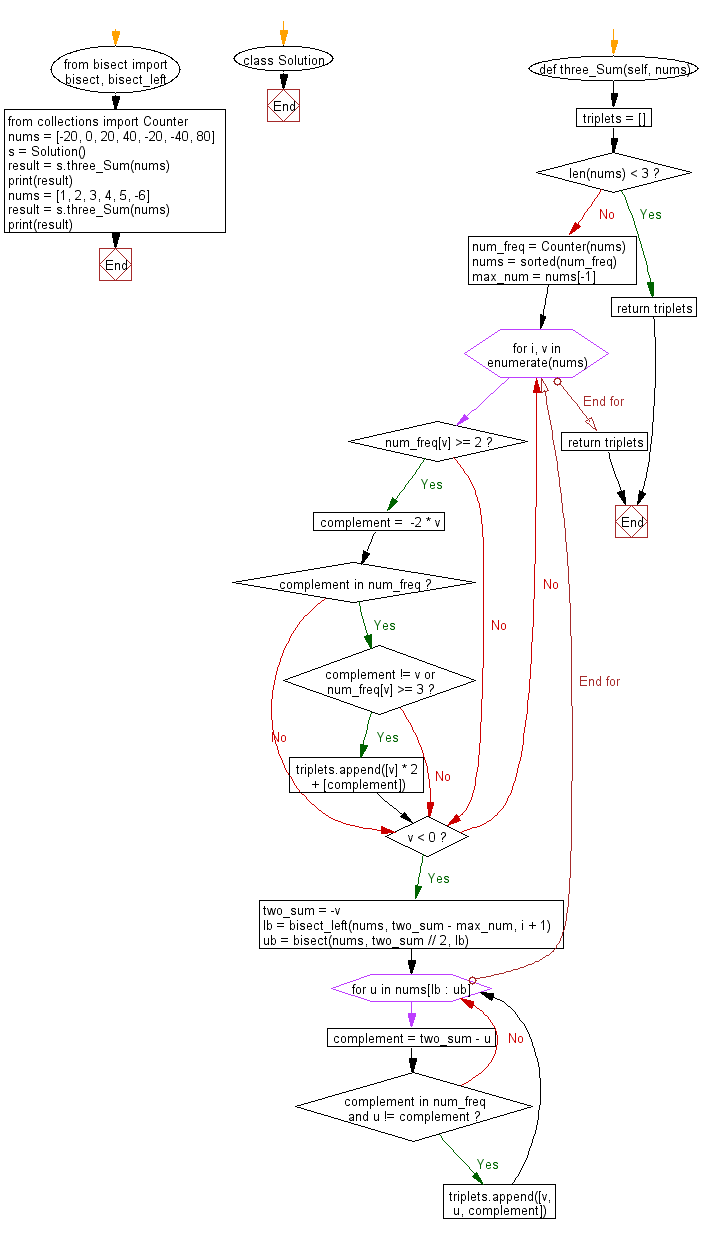﻿ Python Bisect: Find three integers which gives the sum of zero in a given array of integers using Binary Search - w3resource# Python Bisect: Find three integers which gives the sum of zero in a given array of integers using Binary Search

## Python Bisect: Exercise-7 with Solution

Write a Python program to find three integers which gives the sum of zero in a given array of integers using Binary Search (bisect).

Sample Solution:

Python Code:

``````from bisect import bisect, bisect_left
from collections import Counter
class Solution:
def three_Sum(self, nums):
"""
:type nums: List[int]
:rtype: List[List[int]]
"""
triplets = []
if len(nums) < 3:
return triplets
num_freq = Counter(nums)
nums = sorted(num_freq)  # Sorted unique numbers
max_num = nums[-1]
for i, v in enumerate(nums):
if num_freq[v] >= 2:
complement =  -2 * v
if complement in num_freq:
if complement != v or num_freq[v] >= 3:
triplets.append([v] * 2 + [complement])

# When all 3 numbers are different.
if v < 0:  # Only when v is the smallest
two_sum = -v

# Lower/upper bound of the smaller of remainingtwo.
lb = bisect_left(nums, two_sum - max_num, i + 1)
ub = bisect(nums, two_sum // 2, lb)
for u in nums[lb : ub]:
complement = two_sum - u
if complement in num_freq and u != complement:
triplets.append([v, u, complement])
return triplets
nums = [-20, 0, 20, 40, -20, -40, 80]
s = Solution()
result = s.three_Sum(nums)
print(result)
nums = [1, 2, 3, 4, 5, -6]
result = s.three_Sum(nums)
print(result)
```
```

Sample Output:

```[[-40, 0, 40], [-20, -20, 40], [-20, 0, 20]]
[[-6, 1, 5], [-6, 2, 4]]
```

Flowchart:## Visualize Python code execution:

The following tool visualize what the computer is doing step-by-step as it executes the said program:

Python Code Editor:

Next: Write a Python program to find a triplet in an array such that the sum is closest to a given number. Return the sum of the three integers.

What is the difficulty level of this exercise?

Test your Programming skills with w3resource's quiz.

﻿

## Python: Tips of the Day

How to make a chain of function decorators?

```from functools import wraps

def makebold(fn):
@wraps(fn)
def wrapped(*args, **kwargs):
return "<b>" + fn(*args, **kwargs) + "</b>"
return wrapped

def makeitalic(fn):
@wraps(fn)
def wrapped(*args, **kwargs):
return "<i>" + fn(*args, **kwargs) + "</i>"
return wrapped

@makebold
@makeitalic
def hello():
return "hello world"

@makebold
@makeitalic
def log(s):
return s

print hello()        # returns "<b><i>hello world</i></b>"
print hello.__name__ # with functools.wraps() this returns "hello"
print log('hello')   # returns "<b><i>hello</i></b>"
```

Ref: https://bit.ly/3cVz5iw